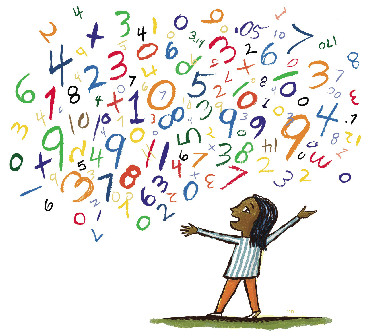* indicates a required field• phone number format invalid• email format invalidProblems detected, please review the form.
Privacy - Terms

# News from Mathnasium of Littleton

### Teaching Number Sense

Nov 24, 2015How can number sense help a child in math?

Quick! What is 2/3 of 240 6/229?

This is the type of problem that can cause a real dread of math. Just the thought of the process and computations involved is enough to make one frustrated and annoyed. Multiplying fractions and mixed numbers! Ugh! Solving this problem will probably take a little division as well. Double ugh!

Yet there is a much simpler and elegant way to solve this without ever putting pen to the paper. All it requires is a little “number sense”.

At the root of number sense are a few basic ideas. One of the most basic and most used ideas in math is the idea of seeing the whole thing and its parts. It occurs throughout math from addition through differential equations and beyond. Let’s see how it applies to the two numbers in our question. First let’s look at 2/3. Fractions tell us two things – how many equal parts the whole is divided into and how many parts to take. So 2/3 says divide the whole into three equal parts and take two of those parts.

Next we can view the mixed number 240 6/229 as a whole thing whose parts are 240 and 6/229. If we want to find two-thirds of a whole thing, we can find two-thirds of each part and add the results together.

If we combine these two ideas we can first find 2/3 of 240 which says divide 240 into three equal parts (each part would be 80) and take two of those parts. This would give us 160. Now divide 6/229 into three parts. This may seem daunting, but consider that if I divide 6 bananas into three equal parts I get 2 bananas in each part. If I divide 6 doughnuts into three equal parts I get 2 doughnuts in each part. So if I divide 6/229 into three equal parts I get 2/229 in each part. And taking two of those parts would be 4/229.

Now we add the parts together and get the final answer: 160 4/229.

Number sense alone can’t be used to solve all problems. However developing number sense in a child along with an understanding of the more general mathematical methods, leads to a deeper understanding and appreciation of math.

Mathnasium’s goal is to build number sense and with it a deep understanding and lifelong appreciation of mathematics. Our programs help students who are at, above, and below grade level to develop number sense as well as the foundational and advanced skills necessary for success in the classroom. Give us a call and find out how Mathnasium of Littleton can help your child 303-979-9077.